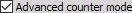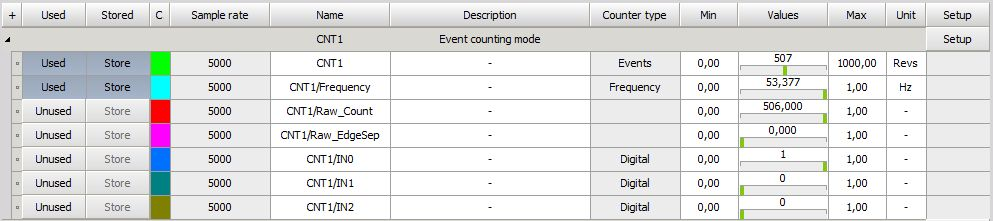• Required hardware - Dewesoft USB
• Setup sample rate - At least 1 kHz

The problem with previous (traditional) counters is that the value of the counter is latched only at a sample rate interval. Therefore we have only discrete values on each sample.

But since the Dewesoft counters can measure where EXACTLY the position of the pulse is in between two samples, we can calculate two things out of this: the exact interpolated position of the counter at the sample point as well as the exact frequency of the pulses.

Dewesoft can perform these measurements on a single counter.

We have to select “Advanced counter mode” in setup: